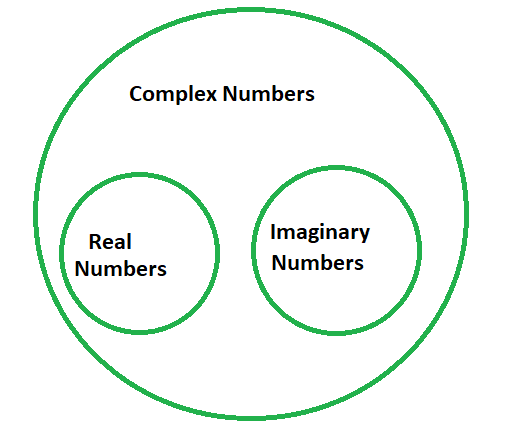# Simplify the Square root of -16 using the imaginary unit i

• Last Updated : 13 Apr, 2022

A complex number is written as a + ib, where a is the real part and ib is the imaginary unit such that i = √-1. Using this logic, 7 + 12i is a complex quantity in which 7 is the real part and 12i – is the imaginary part.### How to Get the Negative Sign Out of Square Root

Suppose a complex number:

C = √-a2

then,

√-a2 = √(-1×a×a) = √(-1)×√(a×a) = i × a = ai

### Question: Simplify the number using the imaginary unit i: Square root of -16.

Given: C = √-16

This can be simplified as:

√-16 = √(-1×4×4)

= √(-1)×√(4×4)

= i × 4

= 4i

### Similar Questions

Question 1: Simplify the number using the imaginary unit i: Square root of -49.

Given: C = √-49

This can be simplified as:

√-49 = √(-1×7×7)

= √(-1)×√(7×7)

= i × 7

= 7i

Question 2: Simplify the number using the imaginary unit i: square root of -512.

Given: C = √-49

This can be simplified as:

√-512 = √(-1×8×8×8)

= √(-1)×√(8×8)×√8

= i × 8 × √(2×2×2)

= i × 8 × 2 × √2

= 16√2i

Question 3: Simplify the number using the imaginary unit i: square root of -100.

Given: C = √-100

This can be simplified as:

√-100 = √(-1×10×10)

= √(-1)×√(10×10)

= i × 10

= 10i

Question 4: Simplify the number using the imaginary unit i: square root of -81.

Given: C = √-81

This can be simplified as:

√-49 = √(-1×9×9)

= √(-1)×√(9×9)

= i × 9

= 9i

Question 5: Simplify the number using the imaginary unit i: square root of -729.

Given: C = √-729

This can be simplified as:

√-729 = √(-1×27×27)

= √(-1)×√(27×27)

= i × 27

= 27i

My Personal Notes arrow_drop_up
Recommended Articles
Page :